# Additive inverse and distance between two points on a number line

##By Don't Memorise

Absolute value of their difference# Fractions On A Number Line | Good To Know | WSKG

##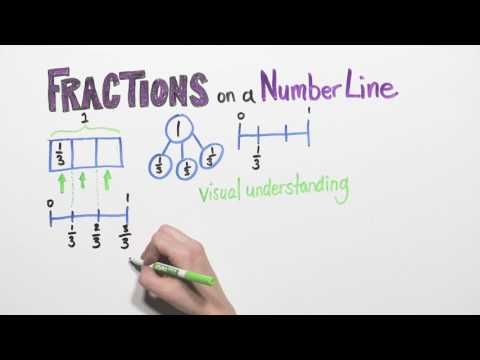By WSKG Public Media

Understand a fraction as a number on the number line; represent fractions on a number line diagram.# Fractions on a Number Line - Mr. Pearson Teaches 3rd Grade

##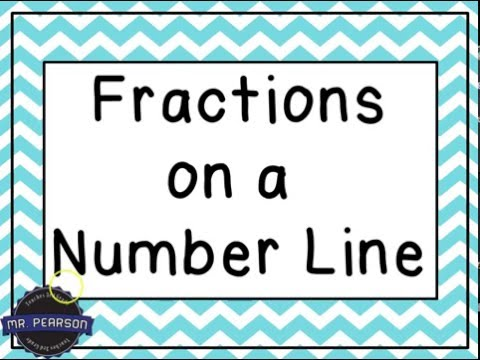By Mr Pearson teaches 3rd grade

This video is designed to teach students how to identify fractions on a number line.# Fractions on a number line | Fractions | 3rd grade | Khan Academy

##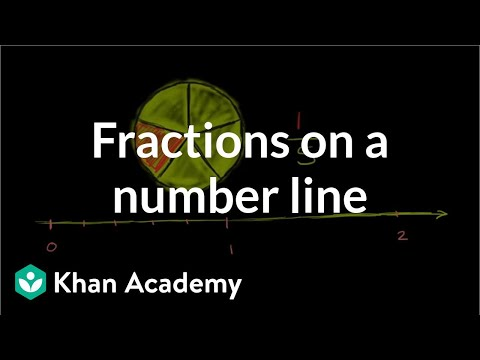By Khan Academy

Graph and locate fractions on a number line.# Comparing fractions on a number line | Fractions | 4th grade | Khan Academy

##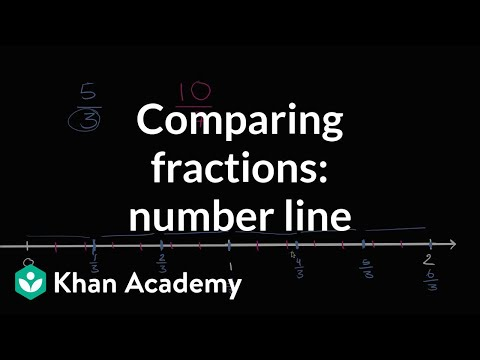By Khan Academy

Comparing fractions on a number line# Equivalent Fractions on a Number Line

##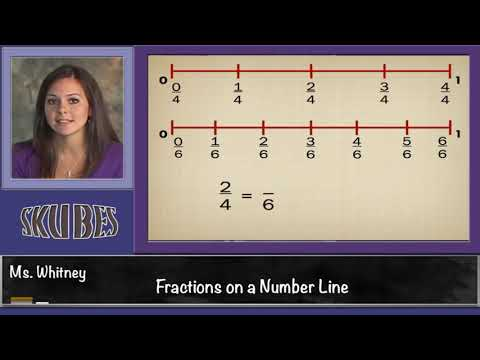By Skubes ed

Explain equivalence of fractions in special cases, and compare fractions by reasoning about their size.# Grade 3 Math #8.5, Fractions on a Number Line

##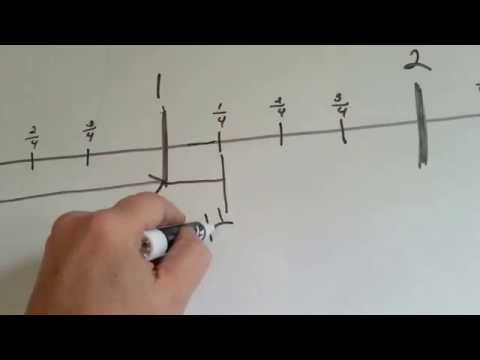By Joann`s School

How fractions are found on a number line. Whole numbers, mixed numbers, fractions on a number line# Plotting Fractions on a Number Line

##By mathlablady4

The instructor first reviews how to plot whole numbers on a number line. She then relates the same concept to fractions. In the video lesson she plots both proper and improper fractions. Very clear instruction and easy for students to visualize the concept.# Inequalities on a number line

##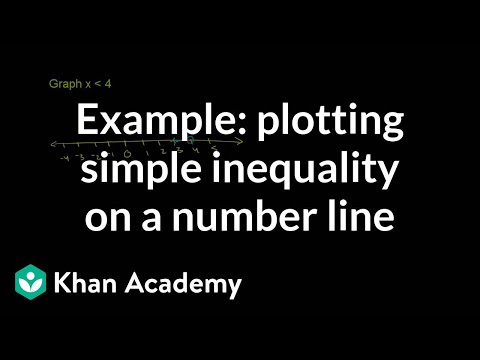By Khan Academy

Learn how to plot a simple inequality on a number line. The example used in this video is x < 4.# ALL OF GRADE 9 MATH IN 60 MINUTES!!! (exam review part 2)

##By Lumos Learning

Here is a great exam review video reviewing all of the main concepts you would have learned in the MPM1D grade 9 academic math course. The video is divided in to 3 parts. This is part 2: Linear Relations. In this video you will review everything there is to know about y=mx+b, scatterplots, and distance time graphs.# 2nd Grade Math Compilation

##By

2nd Grade math is fun with these learning videos! Each lesson can help students with word problems, math games or help prepare for a test! Great for a review of 2nd grade or for an introduction!